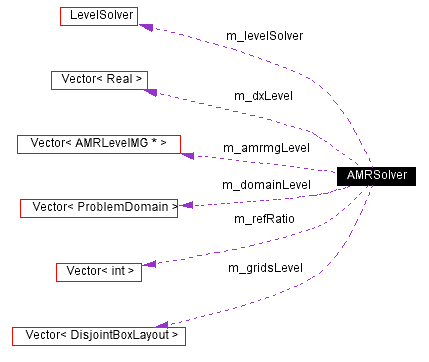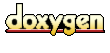Main Page   Namespace List   Class Hierarchy   Alphabetical List   Compound List   File List   Compound Members   File Members

# AMRSolver Class Reference

Class which manages grid hierarchy and composite elliptic solution. More...

#include <AMRSolver.H>

Collaboration diagram for AMRSolver:[legend]
List of all members.

## Public Methods

AMRSolver ()
AMRSolver (const Vector< DisjointBoxLayout > &a_gridsLevel, const Vector< Box > &a_domainLevel, const Vector< Real > &a_dxLevel, const Vector< int > &a_refRatio, int a_numLevels, int a_lBase, const LevelOp *const a_opin, int ncomp=1)
AMRSolver (const Vector< DisjointBoxLayout > &a_gridsLevel, const Vector< ProblemDomain > &a_domainLevel, const Vector< Real > &a_dxLevel, const Vector< int > &a_refRatio, int a_numLevels, int a_lBase, const LevelOp *const a_opin, int ncomp=1)
void define (const Vector< DisjointBoxLayout > &a_gridsLevel, const Vector< Box > &a_domainLevel, const Vector< Real > &a_dxLevel, const Vector< int > &a_refRatio, int a_numLevels, int a_lBase, const LevelOp *const a_opin, int ncomp=1)
void define (const Vector< DisjointBoxLayout > &a_gridsLevel, const Vector< ProblemDomain > &a_domainLevel, const Vector< Real > &a_dxLevel, const Vector< int > &a_refRatio, int a_numLevels, int a_lBase, const LevelOp *const a_opin, int ncomp=1)
~AMRSolver ()
bool isDefined () const
void setNumSmoothUp (int a_numSmoothUp)
void setNumSmoothDown (int a_numSmoothDown)
void setTolerance (Real a_tolerance)
void setOperatorTolerance (Real a_operatorTolerance)
void setMaxIter (int a_maxIter)
void setMinIter (int a_minIter)
void setNumVCyclesBottom (int a_numVCycleBottom)
void solveAMR (Vector< LevelData< FArrayBox > * > &a_phiLevel, const Vector< LevelData< FArrayBox > * > &a_rhsLevel)
Solves on hierarchy to tolerance m_tolerance.

void AMRVCycleMG (Vector< LevelData< FArrayBox > * > &a_corrLevel, const Vector< LevelData< FArrayBox > * > &a_residLevel)
Vector< RealcomputeResidualNorm (Vector< LevelData< FArrayBox > * > &a_phiLevel, const Vector< LevelData< FArrayBox > * > &a_rhsLevel, int normNtype)
Vector< RealcomputeResidualNorm (Vector< LevelData< FArrayBox > * > &a_residLevel, Vector< LevelData< FArrayBox > * > &a_phiLevel, const Vector< LevelData< FArrayBox > * > &a_rhsLevel, int normNtype)
void computeAMRResidual (Vector< LevelData< FArrayBox > * > &a_phiLevel, const Vector< LevelData< FArrayBox > * > &a_rhsLevel, LevelData< FArrayBox > &res, int ilev)
void applyAMROperator (Vector< LevelData< FArrayBox > * > &a_phiLevel, LevelData< FArrayBox > &a_LofPhi, int a_ilev)
void applyAMROperatorHphys (Vector< LevelData< FArrayBox > * > &a_phiLevel, LevelData< FArrayBox > &a_LofPhi, int a_ilev)
void setVerbose (bool a_verbose)

## Protected Methods

void setDefaultValues ()
void clear ()

## Protected Attributes

Real m_errorTolerance
Real m_tolerance
Real m_operatorTolerance
int m_numLevels
int m_finestLevel
Vector< int > m_refRatio
Vector< Realm_dxLevel
Vector< DisjointBoxLayoutm_gridsLevel
Vector< ProblemDomainm_domainLevel
int m_maxIter
int m_minIter
int m_numSmoothUp
int m_numVCyclesBottom
int m_numSmoothDown
Vector< AMRLevelMG * > m_amrmgLevel
bool m_isDefined
LevelSolver m_levelSolver
int m_lBase
int m_ncomp
bool m_verbose

class AMRLevelMG

## Detailed Description

Class which manages grid hierarchy and composite elliptic solution.

AMRSolver manages the AMR/multigrid solution to the elliptic equation on a multiple level grid that satisfies certain proper nesting conditions. It can be used either as a solver, or to apply the AMR / multigrid V-cycle as a preconditioner for some other iterative method, such as a Krylov method.

## Constructor & Destructor Documentation

 AMRSolver::AMRSolver ( )
 Creates a AMRSolver whose internal state is undefined. Need to call define(...) function to use any of the functionality of the class

 AMRSolver::AMRSolver ( const Vector< DisjointBoxLayout > & a_gridsLevel, const Vector< Box > & a_domainLevel, const Vector< Real > & a_dxLevel, const Vector< int > & a_refRatio, int a_numLevels, int a_lBase, const LevelOp *const a_opin, int ncomp = 1 )
 Creates a fully-defined AMRSolver. Calls define function with identical arguments

 AMRSolver::AMRSolver ( const Vector< DisjointBoxLayout > & a_gridsLevel, const Vector< ProblemDomain > & a_domainLevel, const Vector< Real > & a_dxLevel, const Vector< int > & a_refRatio, int a_numLevels, int a_lBase, const LevelOp *const a_opin, int ncomp = 1 )
 Creates a fully-defined AMRSolver. Calls define function with identical arguments

 AMRSolver::~AMRSolver ( )

## Member Function Documentation

 void AMRSolver::AMRVCycleMG ( Vector< LevelData< FArrayBox > * > & a_corrLevel, const Vector< LevelData< FArrayBox > * > & a_residLevel )
 Does one relaxation V-cycle using a MG solver. Problem is assumed to already be in residual-correction form. \ {\bf Inputs:} \ corrLevel - pointers to current guess at the correction values for levels lMin = max(lBase-1,0) ... lMax. Vector index corresponds to level number. \ residLevel - pointers to AMR residual for levels lMin ... lMax. Vector index corresponds to level number. \{\bf Outputs:} \ corrLevel - LevelDatas pointed to for levels lMin,...,lMax are updated in place.

 void AMRSolver::applyAMROperator ( Vector< LevelData< FArrayBox > * > & a_phiLevel, LevelData< FArrayBox > & a_LofPhi, int a_ilev )
 Calculate multilevel L(phi). includes refluxing and all that This is the three-level operator.

 void AMRSolver::applyAMROperatorHphys ( Vector< LevelData< FArrayBox > * > & a_phiLevel, LevelData< FArrayBox > & a_LofPhi, int a_ilev )
 Calculate multilevel L(phi) with homogeneous physical BCs (but with inhomogeneous C/F BCs). includes refluxing and all that This is the three-level operator.

 void AMRSolver::clear ( )  [protected]

 void AMRSolver::computeAMRResidual ( Vector< LevelData< FArrayBox > * > & a_phiLevel, const Vector< LevelData< FArrayBox > * > & a_rhsLevel, LevelData< FArrayBox > & res, int ilev )
 Calculate multilevel residual on level ilev.

 Vector AMRSolver::computeResidualNorm ( Vector< LevelData< FArrayBox > * > & a_residLevel, Vector< LevelData< FArrayBox > * > & a_phiLevel, const Vector< LevelData< FArrayBox > * > & a_rhsLevel, int normNtype )
 Calculate norm of multilevel residual on levels lBase to lmax. Does not include data covered by finer levels. Also returns residual in argument a_residLevel.

 Vector AMRSolver::computeResidualNorm ( Vector< LevelData< FArrayBox > * > & a_phiLevel, const Vector< LevelData< FArrayBox > * > & a_rhsLevel, int normNtype )
 Calculate norm of multilevel residual on levels lBase to lmax. Does not include data covered by finer levels.

 void AMRSolver::define ( const Vector< DisjointBoxLayout > & a_gridsLevel, const Vector< ProblemDomain > & a_domainLevel, const Vector< Real > & a_dxLevel, const Vector< int > & a_refRatio, int a_numLevels, int a_lBase, const LevelOp *const a_opin, int ncomp = 1 )
 Defines AMRSolvers' internal state \ {\bf Inputs:} \ gridsLevel --- The grids at all levels. Each element in the vector is a level's worth of grids. gridsLevel are grids for level 0 and so forth. Vector index corresponds to level number. \ domainLevel --- The domains at all levels. Each element in the vector is a level's domain. domainLevel is the domain for level 0 and so forth. Vector index corresponds to level number. \ dxLevel --- The grid spacing at all levels. Each element in the vector is a level's $\dx$. dxLevel--- is $\dx$ for level 0 and so forth. Vector index corresponds to level number. \ ref_ratio--- The refinement ratio between all levels. ref_ratio is the refinement ratio between level 0 and level 1; Vector index corresponds to level number. \ numlevels The number of AMR levels in the calculation. The length of the Vector~s has to be at least numlevels. \ lBase - coarsest level on which solution is to be computed. This needs to be set at the time of definition, in order to build the bottom LevelSolver. \ opin_a The levelop to use in the solution.

 void AMRSolver::define ( const Vector< DisjointBoxLayout > & a_gridsLevel, const Vector< Box > & a_domainLevel, const Vector< Real > & a_dxLevel, const Vector< int > & a_refRatio, int a_numLevels, int a_lBase, const LevelOp *const a_opin, int ncomp = 1 )
 Defines AMRSolvers' internal state \ {\bf Inputs:} \ gridsLevel --- The grids at all levels. Each element in the vector is a level's worth of grids. gridsLevel are grids for level 0 and so forth. Vector index corresponds to level number. \ domainLevel --- The domains at all levels. Each element in the vector is a level's domain. domainLevel is the domain for level 0 and so forth. Vector index corresponds to level number. \ dxLevel --- The grid spacing at all levels. Each element in the vector is a level's $\dx$. dxLevel--- is $\dx$ for level 0 and so forth. Vector index corresponds to level number. \ ref_ratio--- The refinement ratio between all levels. ref_ratio is the refinement ratio between level 0 and level 1; Vector index corresponds to level number. \ numlevels The number of AMR levels in the calculation. The length of the Vector~s has to be at least numlevels. \ lBase - coarsest level on which solution is to be computed. This needs to be set at the time of definition, in order to build the bottom LevelSolver. \ opin_a The levelop to use in the solution.

 bool AMRSolver::isDefined ( ) const
 Returns true if full define function has been called.

 void AMRSolver::setDefaultValues ( )  [protected]

 void AMRSolver::setMaxIter ( int a_maxIter )
 Set max number of iterations. Default is 42.

 void AMRSolver::setMinIter ( int a_minIter )
 Set min number of iterations. Only relevant when residual is not decreasing fast enough to satisfy the "operator tolerance". Default is 4.

 void AMRSolver::setNumSmoothDown ( int a_numSmoothDown )
 Set number of multigrid smoothings on way down v-cycle; Default is 4.

 void AMRSolver::setNumSmoothUp ( int a_numSmoothUp )
 Set number of multigrid smoothings on way up v-cycle. Default is 4

 void AMRSolver::setNumVCyclesBottom ( int a_numVCycleBottom )
 Set the number of v-cycles performed from the base level to the maximum coarsening of the base level during one overall v-cycle on the AMR hierarchy. Default is 1.

 void AMRSolver::setOperatorTolerance ( Real a_operatorTolerance )
 Set "operator tolerance" of iterative solution. Iteration will stop if (new_residual/old_residual) > 1-operatorTolerance (and at least minIter iterations have been performed). Default is 1.0e-5.

 void AMRSolver::setTolerance ( Real a_tolerance )
 Set tolerance of iterative solution. Default is 1.0e-10.

 void AMRSolver::setVerbose ( bool a_verbose )
 set whether the solver does i/o while solving. default is true

 void AMRSolver::solveAMR ( Vector< LevelData< FArrayBox > * > & a_phiLevel, const Vector< LevelData< FArrayBox > * > & a_rhsLevel )
 Solves on hierarchy to tolerance m_tolerance. Solves the elliptic equation over the hierarchy of levels lBase ... lMax where $lMax = numlevels-1$. If lBase > 0, then the data at level lBase - 1 is used to interpolate boundary conditions at boundary cells that are not adjacent to the domain boundary. \{\bf Inputs:} \ phiLevel - pointers to current guess at the solution values for levels (lMin = max(lBase-1,0)) ... lMax. Vector index corresponds to level number. \ rhsLevel - pointers to right-hand side for levels lmin ... lMax. Vector index corresponds to level number. \{\bf Outputs:} \ phiLevel - LevelDatas pointed to for levels lMin,...,lMax are updated in place.

## Friends And Related Function Documentation

 friend class AMRLevelMG [friend]

## Member Data Documentation

 Vector AMRSolver::m_amrmgLevel [protected]

 Vector AMRSolver::m_domainLevel [protected]

 Vector AMRSolver::m_dxLevel [protected]

 Real AMRSolver::m_errorTolerance [protected]

 int AMRSolver::m_finestLevel [protected]

 Vector AMRSolver::m_gridsLevel [protected]

 bool AMRSolver::m_isDefined [protected]

 int AMRSolver::m_lBase [protected]

 LevelSolver AMRSolver::m_levelSolver [protected]

 int AMRSolver::m_maxIter [protected]

 int AMRSolver::m_minIter [protected]

 int AMRSolver::m_ncomp [protected]

 int AMRSolver::m_numLevels [protected]

 int AMRSolver::m_numSmoothDown [protected]

 int AMRSolver::m_numSmoothUp [protected]

 int AMRSolver::m_numVCyclesBottom [protected]

 Real AMRSolver::m_operatorTolerance [protected]

 Vector AMRSolver::m_refRatio [protected]

 Real AMRSolver::m_tolerance [protected]

 bool AMRSolver::m_verbose [protected]

The documentation for this class was generated from the following file:
Generated on Thu Aug 29 11:07:35 2002 for Chombo&INS by1.2.16